English

MQL4 BookVariablesArrays

# Arrays

A large part of information processed by application programs is contained in arrays.

## Concept of Arrays

Array is an arranged set of values of one-type variables that have a common name. Arrays can be one-dimensional and multidimensional. The maximum admissible amount of dimensions in an array is four. Arrays of any data types are allowed.

Array element is a part of an array; it is an indexed variable having the same name and some value.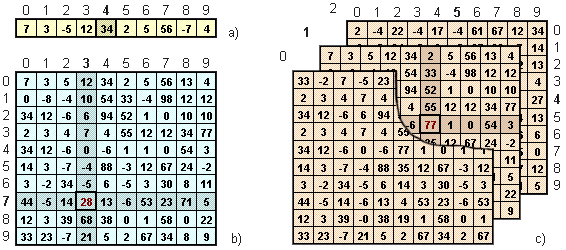Fig. 59. Graphical presentation of arrays of integer type: a) one-dimensional; b) two-dimensional; c) three-dimensional.

## Indexing

Array element index is one or several integer values indicated in the form of a constant, variable or expression enumerated comma-separated in square brackets. Array element index uniquely defines place of an element in an array. Array element index is indicated after a variable identifier (array name) and is an integral part of an array element. In MQL4 indexing starting form zero is used.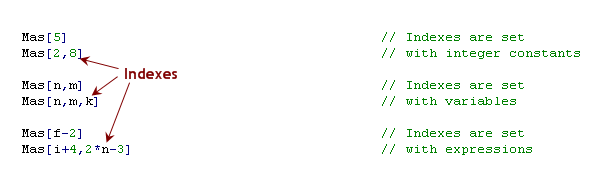The way of specifying indexes when each index is in square brackets is also acceptable: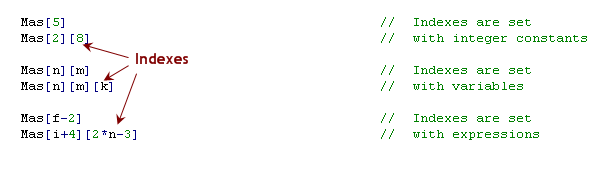The closest everyday analogue of a two-dimensional array is a cinema hall. The row number is the first index value, the number of place in a raw is the value of the second index, viewers are array elements, viewer's surname is the value of an array element, cinema ticket (specifying row and place) is a method to access the value of an array element.

Before using an array in a program, it must be declared. An array can be declared like a variable on the global and local level. Accordingly, values of global array elements are available to the whole program, values of a local one - only to the function, in which it is declared. An array cannot be declared on the level of a client terminal, that is why global variables of client terminal cannot be gathered into an array. Array elements values can be of any type. Values of all array elements are of the same type, namely of the type indicated at array declaration. When declaring an array, data type, array name and number of elements of each dimension must be specified: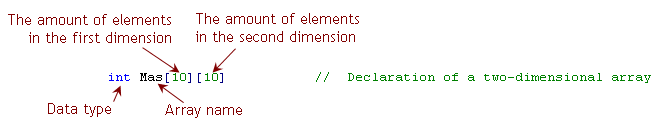Access to array elements is implemented elementwise, i.e. at a moment of time only one component can be accessed. Type of array component value is not specified in the program. Array component value can be assigned or changed using the assignment operator: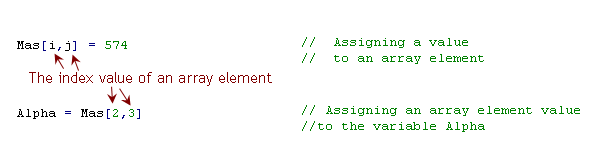The value of array elements in Fig. 59 are the following:

- for a one-dimensional, array Mas element value is integer 34;

- for two-dimensional array, Mas[3,7] element value is integer 28;

- for three-dimensional array, Mas[5,4,1] element value is integer 77.Note: minimal value of array element index is 0 (zero) and maximal value is smaller by one than the number of elements in a corresponding dimension indicated at array declaration.

For example, for the array Mas the element with the smallest indexes value is the element Mas[0,0], the one with maximal indexes values is the element Mas[9,14] .

Operations with arrays can also be conducted using standard functions. For more information, please refer to documentation on the developer's website (https://docs.mql4.com) or to "Help" in MetaEditor. Some of these functions are analyzed further.

## Array Initialization

An array can be initialized only by constants of a corresponding type. One-dimensional and multi-dimensional arrays are initialized by one-dimensional sequence of constants separated by commas. The sequence is included into curly brackets:

`int Mas_i = { 0, 1, 2, 3,   10, 11, 12, 13,   20, 21, 22, 23 }; double Mas_d = { 0.1, 0.2, -0.3,    -10.2, 1.5, 7.0 }; bool Mas_b = { false, true, false, true, true }`

In the initialized sequence one or several constants can be omitted. In such a case corresponding array elements of numeric type are initialized by zero, elements of arrays of string type are initialized by string value "" (quotation marks without a space), i.e by an empty line (shouldn't be confused with a space). The next program displays values of arrays, initialized by a sequence with omission of some values (script arrayalert.mq4):

`//--------------------------------------------------------------------// arrayalert.mq4// The code should be used for educational purpose only.//--------------------------------------------------------------------int start()                                     // Special funct. start()  {   string Mas_s = {"a","b", ,"d"};           // String array   int Mas_i = { 0,1,2, ,4,5 };              // Integer type array   Alert(Mas_s,Mas_s,Mas_s,Mas_s);  // Displaying   Alert(Mas_i,Mas_i,Mas_i,Mas_i,Mas_i,Mas_i);   return;                                      // Exit start()  }//--------------------------------------------------------------------`

If the size of a one-dimension initialized array is not specified, it is defined by a compiler based on the initialized sequence. An array can be also initialized by the standard function ArrayInitialize(). All arrays are static, i.e. are of static type even if at the initialization this is not explicitly indicated. It means all arrays preserve their values between calls of the function, in which the array is declared (see Types of Variables).

All arrays used in MQL4 can be divided into two groups: user-defined arrays (created by a programmer's initiative) and arrays-timeseries (arrays with predefined names and data types). Defining sizes of user-defined arrays and values of their elements depends on how a program is created and, ultimately, on a programmer's will. Values of user-defined array elements are preserved during the whole program execution time and can be changed after calculations. However, values of elements in arrays-timeseries cannot be changed, their size can be increased when the history is updated.

## User-Defined Arrays

In the section Operator 'switch' we analyzed Problem 18. Let's make it more complicated (increase the number of points written in words to 100) and find the solution using arrays.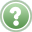Problem 25. Create a program, in which the following conditions are implemented: if price exceeds a certain level, display a message, in which the excess is indicated (up to 100 points); in other cases inform that the price does not exceed this level..

Solution of Problem 25 using a string array can be the following (Expert Advisor stringarray.mq4):

`//--------------------------------------------------------------------// stringarray.mq4// The code should be used for educational purpose only.//--------------------------------------------------------------------extern double Level=1.3200;                     // Preset level string Text;                               // Array declaration//--------------------------------------------------------------------int init()                                      // Special funct. init()  {                                             // Assigning values   Text="one ";             Text="fifteen ";   Text="two ";             Text="sixteen ";   Text="three ";           Text="seventeen ";   Text="four ";            Text="eighteen ";   Text="five ";            Text="nineteen ";   Text="six ";             Text="twenty ";   Text="seven ";           Text="thirty ";   Text="eight ";           Text="forty ";   Text="nine ";            Text="fifty ";   Text="ten ";            Text="sixty";   Text="eleven ";         Text="seventy ";   Text="twelve ";         Text="eighty ";   Text="thirteen ";       Text="ninety";   Text="fourteen ";       Text= "hundred";   // Calculating values   for(int i=20; i<=90; i=i+10)                // Cycle for tens     {      for(int j=1; j<=9; j++)                  // Cycle for units         Text[i+j]=Text[i] + Text[j];          // Calculating value        }   return;                                     // Exit init()  }//--------------------------------------------------------------------int start()                                     // Special funct. start()  {   int Delta=NormalizeDouble((Bid-Level)/Point,0);// Excess //--------------------------------------------------------------------   if (Delta>=0)                                // Price is not higher than level     {      Alert("Price below level");               // Alert      return;                                   // Exit start()     }//--------------------------------------------------------------------   if (Delta<100)                               // Price higher than 100     {      Alert("More than hundred points");        // Alert      return;                                   // Exit start()     }//--------------------------------------------------------------------   Alert("Plus ",Text[Delta],"pt.");            // Displaying   return;                                      // Exit start()  }//--------------------------------------------------------------------`

In the problem solution string array Text[] is used. During the program execution values of array elements are not changed. The array is declared on a global level (outside special functions), the initial array fulfillment is done in init(). Thus in the special function start() only calculations necessary at each tick are conducted.

To some part of Text[] array elements values of string constants are assigned. To another part values calculated in included cycles by summing lines are assigned.

`   for (int i = 20; i<=90; i=i+10)              // Cycle for tens     {      for (int j=1; j<=9; j++)                  // Cycle for units         Text[i+j] = Text[i] + Text[j];         // Calculating value        }`

The meaning of these calculations can be easily understood: for each array element with the index from 21 to 99 (except indexes multiple of 10) corresponding string values are calculated. Pay attention to values of indexes specified in the lines:

`         Text[i+j] = Text[i] + Text[j];         // Calculating value`

As index values variables (the values of which are changed in the cycle) and expressions are used. Depending on values of variables i and j, the program will refer to the corresponding Text[] array elements, sum up their values and assign the result to an array element with the index, the value of which is calculated (i+j). For example, if at some calculation stage the value of i is equal to 30 and that of j is equal to 7, the name of elements whose values are summed up are correspondingly Text and Text, name of the element, to which the result is assigned will be Text. Any other variable of integer type can be used as the value of ab array element. In this example in the function start() element name of the same array with the index Delta is used - Text[Delta].

The special function start() has a simple code. Calculations are conducted depending on the value of Delta. If it is smaller or equal to 100 points, after the corresponding message is shown start() execution finishes. If the value is within the specified range, an alert is shown according to the problem conditions.Fig. 60. Displaying desired values by the EA stringarray.mq4.

Pay attention to the solution of Problem 18. If we used the same solution for Problem 25, operator 'switch' would contain about 100 lines - one line for each solution variant. Such an approach to program development cannot be considered satisfactory. Moreover, such solutions are useless if we need to process tens and sometimes hundreds of thousand variable values. In such cases use of arrays is justified and very convenient.

## Arrays-Timeseries

Array-timeseries is an array with a predefined name (Open, Close, High, Low, Volume or Time), the elements of which contain values of corresponding characteristics of historic bars.

Data contained in arrays-timseries carry a very important information and are widely used in programming in MQL4. Each array-timeseries is a one-dimensional array and contains historic data about one certain bar characteristic. Each bar is characterized by an opening price Open[], closing price Close[], maximal price High[], minimal price Low[], volume Volume[] and opening time Time[]. For example, array-timeseries Open[] carries information about opening prices of all bars present in a security window: the value of array element Open is the opening price of the first bar, Open is the opening price of the second bar, etc. The same is true for other timeseries.

Zero bar is a current bar that has not fully formed yet. In a chart window the zero bar is the last right one.

Bars (and corresponding indexes of arrays-timeseries) counting is started from the zero bar. The values of array-timeseries elements with the index  are values that characterize the zero bar. For example, the value of Open is the opening price of a zero bar. Fig. 61 shows numeration of bars and bar characteristics (reflected in a chart window when a mouse cursor is moved to a picture).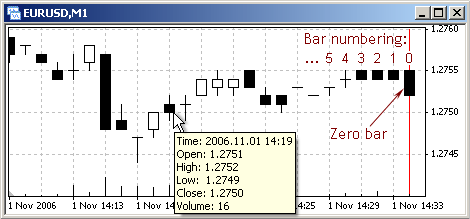Fig. 61. Each bar is characterized by a set of values contained in arrays-timeseries.
Bars counting starts from a zero bar.

Zero bar in Fig. 61 has the following characteristics:

 Index Open[] Close[] High[], Low[], Time[]  1.2755 1.2752 1.2755 1.2752 2006.11.01 14:34

After some time the current bar will be formed and a new bar will appear in a security window. Now this new bar will be a zero bar and the one hat has just formed will become the first one (with the index 1):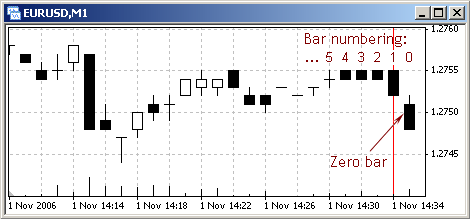Fig. 62. Bars are shifted after some time, while numeration is not shifted.

Now the values of arrays-timeseries elements will be the following:

 Index Open[] Close[] High[], Low[], Time[]  1.2751 1.2748 1.2752 1.2748 2006.11.01 14:35  1.2755 1.2752 1.2755 1.2752 2006.11.01 14:34

Further in the security window new bars will appear. The current yet unformed the rightest bar will always be zero, the one to the left of it will be the first bar, the next one - the second, etc. However, a bar's characteristics are not changed: the bar in the example was opened at 14:43, and his opening price will still remain 14:43, its other parameters will also remain unchanged. However, the index of this bar will increase after the appearance of new bars.

So, the most important feature concerning arrays-timeseries is the following: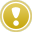Values of array-timeseries elements are a bar's proper characteristics and are nether changed (except the following characteristics of a zero bar: Close, High, Low, Volume ), a bar's index reflects its deepening into future for the current moment and is changed in course of time.

It should be also noted the bar opening time is counted multiple of calender minutes, seconds are not taken into account. In other words, if in the period between 14:34 and 14:35 a new tick has come at 14:34:07, a new bar with opening time 14:43 will appear in the minute timeframe. Accordingly, bar opening time in the 15-minute timeframe is multiple of 15 minutes, within an hour interval the first bar is opened at n hours 00 minutes, the second one at n:15, the third - at n:30, the fourth - at n:45.

To understand correctly the significance of indexes in timeseries, let's solve a simple problem:Problem 26. Find the minimal and the maximal price among the last n bars.

Pay attention, solution of such problems is impossible without referring to values of arrays-timeseries. An Expert Advisor defining the maximal and the minimal price among the preset number of last bars may have the following solution (extremumprice.mq4):

`//--------------------------------------------------------------------// extremumprice.mq4 // The code should be used for educational purpose only.//--------------------------------------------------------------------extern int Quant_Bars=30;                       // Amount of bars//--------------------------------------------------------------------int start()                                     // Special funct. start()  {   int i;                                       // Bar number    double Minimum=Bid,                          // Minimal price          Maximum=Bid;                          // Maximal price    for(i=0;i<=Quant_Bars-1;i++)                 // From zero (!) to..     {                                          // ..Quant_Bars-1 (!)      if (Low[i]< Minimum)                      // If < than known         Minimum=Low[i];                        // it will be min      if (High[i]> Maximum)                     // If > than known         Maximum=High[i];                       // it will be max     }   Alert("For the last ",Quant_Bars,            // Show message           " bars Min= ",Minimum," Max= ",Maximum);   return;                                      // Exit start()  }//--------------------------------------------------------------------`

In the program extremumprice.mq4 a simple algorithm is used. Amount of bars to be analyzed is set up in the external variable Quant_Bars. At the program beginning current price value is assigned to the desired Minimum and Maximum. The search of the maximal and minimal values is conducted in the cycle operator:

`   for(i=0;i<=Quant_Bars-1;i++)                 // From zero (!) to..     {                                         // ..Quant_Bars-1 (!)      if (Low[i]< Minimum)                      // If < than known         Minimum = Low[i];                      // it will be min      if (High[i]> Maximum)                     // If > than known         Maximum = High[i];                     // it will be max     }`

Here descriptive is the interval of index values (integer variable i) of processed array-timeseries elements Low[i] and High[i]. Pay attention to the Expression_1 and Condition in the cycle operator header:

`   for(i=0;i<=Quant_Bars-1;i++)                 // From zero (!) to..`

In the first iteration calculations are conducted with zero index values. It means in the first iteration the values of the zero bar are analyzed. Thus it is guaranteed that the last price values that appeared in a security window are also taken into account. The section Predefined Variables contains the rule according to which values of all predefined variables including arrays-timeseries are updated at the moment of special functions' start. So, none of price values will remain uncounted.

The list index of timeseries elements processed in a cycle is the index smaller by one than the number of processed bars. In our example the number of bars is 30. It means the maximal index value must be 29. So, values of timeseries elements with indexes from 0 to 29, i.e. for 30 bars, will be processed in the cycle.

It is easy to understand the meaning of calculations in the cycle operator body:

`      if (Low[i]< Minimum)                      // If < than known         Minimum = Low[i];                      // it will be min      if (High[i]> Maximum)                     // If > than known         Maximum = High[i];                     // it will be max`

If the current value Low[i] (i.e. during the current iteration with the current index value) is lower than the known minimal value, it becomes the new minimal value. The same way the maximal value is calculated. By the moment of cycle end, variables Minimum and Maximum get the desired value. In further lines these values are displayed.

Launching this program one can get a result like this: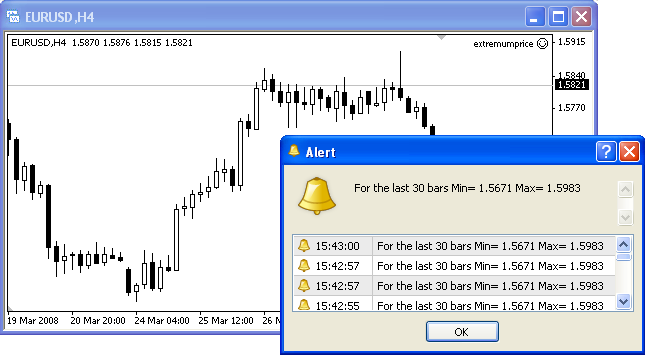Fig. 63. Result of the EA extremumprice.mq4 operation.

Pay attention that the Expert Advisor may operate infinitely long showing correct results, and the program will use the same index values (in this case from 0 to 29). Values of arrays-timeseries elements with the zero index will change at the moment of a new appearance and values of arrays-timeseries elements characterizing the zero bar may change at any next tick (except values of Open[] and Time[] that do not change on the zero bar).

In some cases it is needed to perform some actions starting from the moment when a bar has been fully formed. This is important, for example, for the implementation of algorithms based on a candlestick analysis. In such cases usually only fully formed bars are taken into account.Problem 27. At the beginning of each bar show a message with the minimal and maximal price among the last n formed bars.

To solve the task, it is necessary to define the fact a new bar beginning, i.e. to detect a new tick on a zero bar. There is a simple and reliable way to do this - analyzing the opening time of a zero bar. Opening time of a zero bar is the bar characteristic that does not change during the bar's formation. New ticks coming during a bar's formation can change its High, Close and Volume. But such characteristics as Open and Time are not changed.

That is why it is enough to remember the opening price of a zero bar and at each tick compare it to the last known zero bar opening price. As soon as a mismatch is found, this will mean the formation of a new bar (and completion of the previous one). In the EA newbar.mq4 the algorithm of detecting a new bar is implemented in the form of a user-defined function:

`//--------------------------------------------------------------------// newbar.mq4  // The code should be used for educational purpose only.//--------------------------------------------------------------------extern int Quant_Bars=15;                       // Amount of barsbool New_Bar=false;                             // Flag of a new bar//--------------------------------------------------------------------int start()                                     // Special funct. start()  {   double Minimum,                              // Minimal price          Maximum;                              // Maximal price//--------------------------------------------------------------------   Fun_New_Bar();                               // Function call   if (New_Bar==false)                          // If bar is not new..      return;                                   // ..return//--------------------------------------------------------------------   int Ind_max =ArrayMaximum(High,Quant_Bars,1);// Bar index of max. price    int Ind_min =ArrayMinimum(Low, Quant_Bars,1);// Bar index of min. price    Maximum=High[Ind_max];                       // Desired max. price   Minimum=Low[Ind_min];                        // Desired min. price   Alert("For the last ",Quant_Bars,            // Show message     " bars Min= ",Minimum," Max= ",Maximum);   return;                                      // Exit start()  }//--------------------------------------------------------------------void Fun_New_Bar()                              // Funct. detecting ..  {                                             // .. a new bar   static datetime New_Time=0;                  // Time of the current bar   New_Bar=false;                               // No new bar   if(New_Time!=Time)                        // Compare time     {      New_Time=Time;                         // Now time is so      New_Bar=true;                             // A new bar detected     }  }//--------------------------------------------------------------------`

Global variable New_Bar is used in the program. If its value is 'true', it means the last known tick is the first tick of a new bar. If New_Bar value is false. the last known tick has appeared within the formation of the current zero bar.

Flag is a variable, the value of which is defined in accordance with some events or facts.

Use of flags in a program is very convenient. The flag value can be defined in one place and used in different places. Sometimes an algorithm is used in the program, in which a decision is made depending on the combination of values of different flags. In the Expert Advisor newbar.mq4 the variable New_Bar is used as a flag. Its value depends directly on the fact of a new bar formation.

Calculations regarding the detection of a new bar are concentrated in the user-defined function Fun_New_Bar(). In the first lines of the function the static variable New_Time is defined (remember, static variables do not loose their values after a function execution is over). At each function call, the value of the global variable New_Bar is set 'false'. The detection of a new bar is performed in 'if' operator:

`   if(New_Time != Time)                      // Compare time     {      New_Time = Time;                       // Now time is so      New_Bar = true;                           // A new bar detected     }`

If New_Time value (calculated in the previous history) is not equal to Time of a zero bar, it denotes the fact of a new bar's formation. In such a case control is passed to 'if' operator body, where the new opening time of the zero bar is remembered and the true value is assigned to New_Bar (for convenience it can be said that the flag is set in an upward position).

When solving such problems, it is important to take into account the peculiarity of using different flags. In this case the peculiarity is that New_Bar value (flag position) must be updated earlier than it will be used in calculation (in this case - in the special function start()). New_Bar value is defined in the user-defined function, that is why it must be called possibly early in the program, i.e. before first calculations, in which New_Bar is used. The special function start() is constructed correspondingly: user-defined function call is performed immediately after the declaration of variables.

The calculation of desired values is worthwhile only if start() is launched by a tick at which the new bar is formed. That's why in start(), immediately after detecting a new bar formation, the flag position (New_Bar value) is analyzed:

`   if (New_Bar == false)                        // If bar is not new..      return;                                   // ..return`

If the last tick that started the execution of start() has not form a new bar, control is passed to an operator that finishes the execution of start(). And only if a new bar has been formed, control is passed to the next lines for the calculation of desired values (what is required by the problem provisions).

Calculation of the maximal and minimal value is performed using standard functions ArrayMaximum() and ArrayMinimum(). Each of the functions returns array element index (of the maximal and minimal value correspondingly) for a specified indexes interval. Under the problem conditions, only completely formed bars must be analyzed, that's why the chosen boundary index values are 1 and Quant_Bars - set amount of bars (the zero bar is not formed yet, i.e. is not taken into account during calculations). For more detailed information about the operation of these and other timeseries accessing functions, refer to the documentation on the developper's website (https://docs.mql4.com) or to "Help" in MetaEditor.

Fig. 64 shows the change of maximal and minimal prices in the preset interval during the program execution: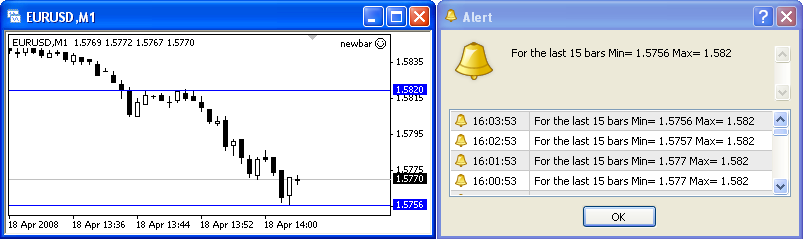Fig. 64. Expert Advisor newbar.mq4 operation result.GlobalVariablesProgramming in MQL4